Sieve Analysis

Sieve analysis is the method of particle size analysis, using which we determine the amount of particles of different sizes present in the soil sample.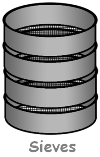Sieve analysis is done for coarse grained soils. In this method soil is sieved through a set of sieves.Sieves are wire screens having square openings. Size of these openings gives the sieves their name which is called Sieve number. A sieve with a mesh opening of 4.75 mm is designated as 4.75 mm Sieve (Sieve number written on sieve).

Similarly a 600 micron sieve refers to a sieve which has a mesh opening of 0.600 mm.

Sieve analysis itself is done in two ways…

Dry Sieve analysis

Wet Sieve analysis

We take roughly 500 gm of soil sample. It should not contain any lumps into it, and If it does, then lumps should be pulverized. We take its weight and say this weight as capital W.

Now sample is sieved through 4.75 mm sieve.The portion of soil, which contains particle size bigger than 4.75 mm is retained on the sieve. This portion is called gravel fraction.

The portion of soil, which contains particle size less than 4.75 mm passed through the sieve. This portion is called sand fraction.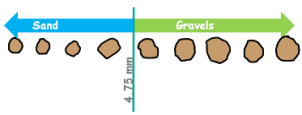This way coarse grained soils are divided into two categories.

1. Gravels, having grain size greater than 4.75 mm and

2. Sand, having grain size smaller than 4.75 mm.

So for sieve analysis of our sample we will need two set of sieves, one for gravel fractions and another for sand fractions.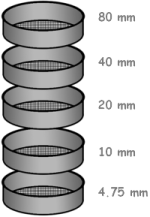For gravel fractions we require sieves of sizes 80 mm, 40 mm, 20 mm, 10 mm and 4.75 mm. This set is called set of coarse sieves as it sieves coarser part of the coarse soils.

Second set of sieves for sand fractions consists sieves of sizes 2 mm, 1 mm, 600 µ, 425 µ, 212 µ, 150 µ and 75 µ. If we didn’t mention then 1 mm that is millimeter is equal to 1000 micrometer which we denote as letter micron. So 1 mm is equal to 1000 micron. So here 600 micron is actually 0.6 mm.

This set of sieves is called set of fine sieves as it sieves finer part of the coarse soils.

The sieves are stacked one over the other with increasing size of their mesh openings from bottom to top. Which means the sieve of largest opening is kept at the top.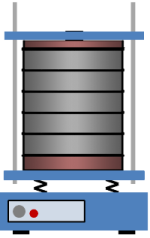A pan which has no opening is placed below the bottom most sieve. The soil sample is placed in the top sieve and a lid to cover this arrangement is placed over the top most sieve.

The whole arrangement is then placed on a mechanical shaker and is shaked for 10 minutes.

Then arrangement is taken out and the amount of soil retained on each sieve and pan is weighed with the accuracy of 0.1 gm.

Let’s say the weights retained on the sieves are w1, w2, w3, w4, w5 and wPan.

Then we calculate the percentage of amount of soil retained on each sieve as weight of retained soil on the sieve divided by total weight of soil sample multiplied by 100.This way we know what percentage of the total weight is retained on a particular sieve.

Percentage weight retained on the 1st sieves is w1 divided by W multiply by 100.Let’s say these percentages as P1, P2, P3, P4, P5 and Ppan.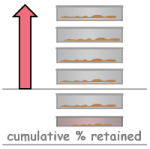For calculation purposes and to determine some important characteristics, we also calculate two values.

First one is cumulative percentage retained.

Now cumulative percentage retained of any sieve is percentage weight retained on that sieve Plus all percentage weight retained on all the sieves above it.

Cumulative percentage retained is the total percentage amount of soil which could not pass the particular sieve.

In other words this percent amount of soil has grain size greater than the sieve number.

So let’s calculate all the cumulative percentage retained for each sieve.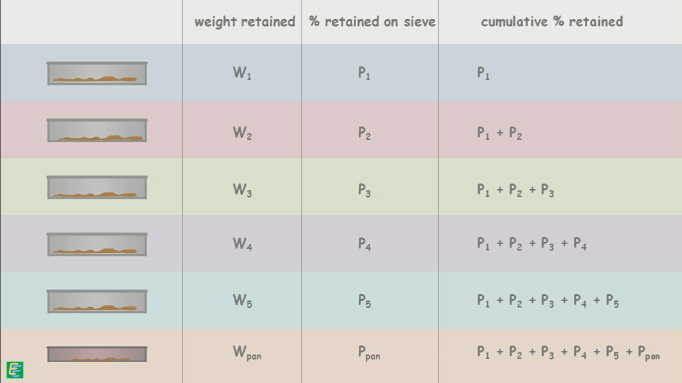Second value we calculate is percentage Finer, using which we plot a graph called particle size distribution curve.

Percentage finer, as name suggests, is the percentage amount of soil which is finer, than a particular sieve.

Consider any sieve from the stack(shown as darker sieve - weight retained on this sieve is W4), now the amount of soil above this sieve is coarser and its total weight in percentage of total soil sample weight is cumulative % retained on this sieve.

And the amount of soil below this sieve is finer portion of the sample which has particle size smaller than openings of this sieve. Total weight of this portion in percentage of total soil sample is % finer of this sieve.This can be easily calculated by simply removing cumulative percentage from the 100 % of soil sample.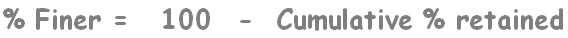Now we can complete our tableThe whole procedure we discussed is Dry Sieve analysis.

The finest sieve used in the analysis is 75µ. If the portion passing through 75µ sieve, which we collect in the pan, is substantial, say 5 to 10%, then wet sieve analysis is carried out.

The purpose of the wet sieve analysis is to remove all the particles which are finer than 75 micron particles from the sample so that we arrive at the correct sieve analysis results.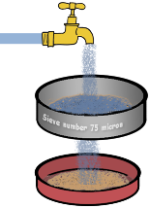The sample is first washed over the 75 µ sieve to remove the fine particles sticking to the sand particles.

The wet sand retained on the 75 µ sieve is dried in an oven and after drying the dry sieve analysis is carried out in the usual manner.

The finer fraction is utilized for the hydrometer or pipette analysis.

Take a look at an example of sieve analysis.

Tags : Sieve

Published on :2019-09-06